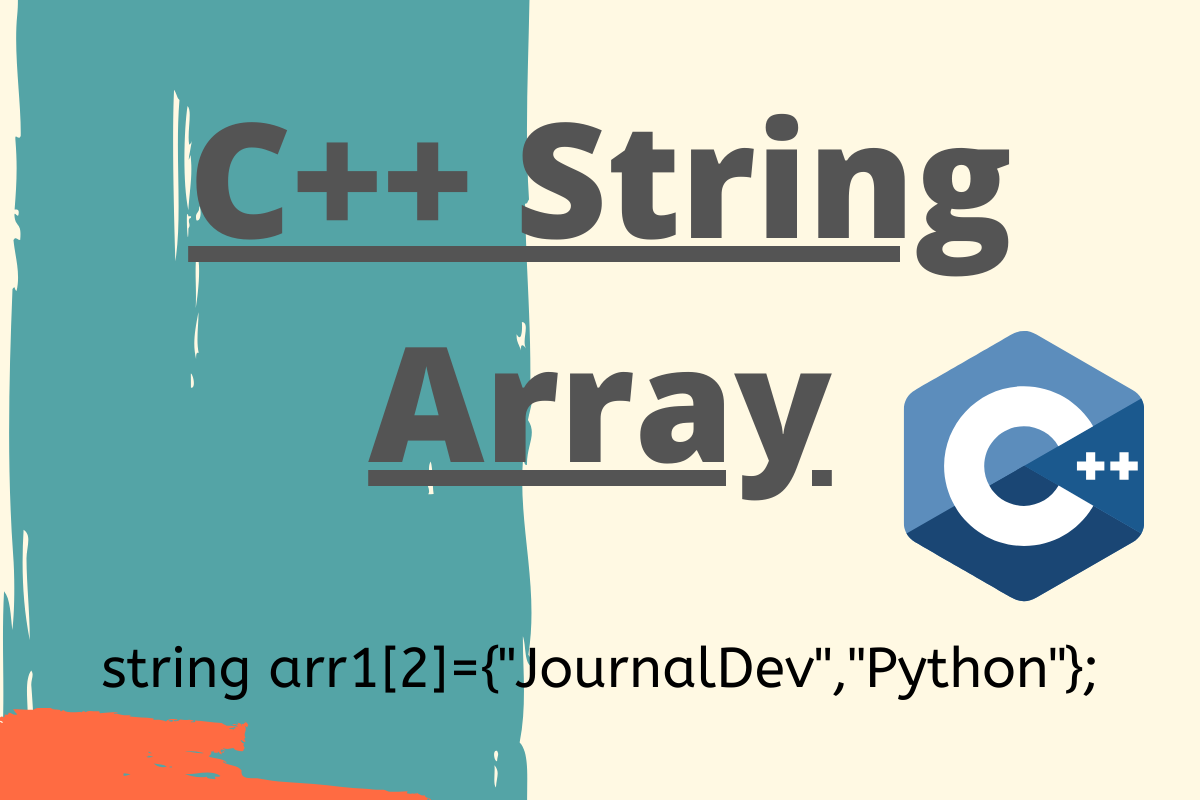# Understanding C++ String Array

Published on August 3, 2022By Safa Mulani
Developer and author at DigitalOcean.While we believe that this content benefits our community, we have not yet thoroughly reviewed it. If you have any suggestions for improvements, please let us know by clicking the “report an issue“ button at the bottom of the tutorial.

Hey, Folks! So, as programmers, we often deal with Arrays of all data types. We’ll cover C++ string array in today’s article.

## Ways to declare an C++ String Array

### 1. The String keyword to Create String Array in C++

C++ provides us with ‘string’ keyword to declare and manipulate data in a String array.

The `string keyword` allocates memory to the elements of the array at dynamic or run-time accordingly. Thus it saves the headache of static memory allocation of data-elements.

Syntax: To declare an array of string using ‘string’ keyword

``````string array-name[size];
``````

Further, we can initialize the array of string using the below syntax:

``````string array-name[size]={'val1','val2',.....,'valN'};
``````

Example 1:

``````#include <bits/stdc++.h>
using namespace std;

int main()
{
string fruits = { "Grapes", "Apple","Pineapple", "Banana", "Jackfruit" };

cout<<"String array:\n";
for (int x = 0; x< 5; x++)
cout << fruits[x] << "\n";
}

``````

In the above example, we have initialized the string array and have used C++ for loops to traverse through the array and print the data items present in the string array.

Output:

``````String array:
Grapes
Apple
Pineapple
Banana
Jackfruit
``````

Example 2:

``````#include <bits/stdc++.h>
using namespace std;

int main()
{

string arr;
cout<<"Enter the elements:"<<endl;
for(int x = 0; x<5;x++)
{
cin>>arr[x];
}

cout<<"\nString array:\n";
for (int x = 0; x< 5; x++)
cout << arr[x] << "\n";
}

``````

As you all can witness, in the above example, we did accept the data items of the string array from the console i.e. user input is taken and we have printed the elements of the string array.

Output:

``````Enter the elements:
Jim
Nick
Daisy
Joha
Sam

String array:
Jim
Nick
Daisy
Joha
Sam
``````

### 2. Using C++ STL Container - Vector

C++ Standard Template Library provides us with containers to work with data and store it efficiently.

Vector, being one such container, stores the array elements in a dynamic manner. Thus, C++ Vectors can be used to create a string array and manipulate the same easily.

Syntax:

``````vector<string>array-name;
``````
• The `vector.push_back(element)` method is used to add elements to the vector string array.
• The `vector.size()` method is used to calculate the length of the array i.e. the count of the elements input to the string array.

Example:

``````#include <bits/stdc++.h>
using namespace std;

int main()
{

vector<string> arr;
arr.push_back("Ace");
arr.push_back("King");
arr.push_back("Queen");

int size = arr.size();
cout<<"Elements of the vector array:"<<endl;
for (int x= 0; x< size; x++)
cout << arr[x] << "\n";
}

``````

Output:

``````Elements of the vector array:
Ace
King
Queen
``````

### 3. Using 2D char array

A 2D array represents an array of string in C++. So, we can use a 2D char array to represent string type elements in an array.

The char array creates and stores elements at static or compile-time i.e. the number and size of elements stay fixed/constant.

Syntax:

``````char array-name[number-of-items][maximun_size-of-string];
``````

Example:

``````#include <bits/stdc++.h>
using namespace std;

int main()
{

char fruits = { "Grapes", "Apple","Pineapple", "Banana", "Jackfruit" };

cout<<"Character array:\n";
for (int x = 0; x< 5; x++)
cout << fruits[x] << "\n";
}

``````

In the above snippet of code, we have created a char array to store string type elements. i.e. char array. Here 5 depicts the count of string elements and 10 points to the maximum size of the input string.

Output:

``````Character array:
Grapes
Apple
Pineapple
Banana
Jackfruit
``````

## C++ String Array as an Argument to a Function

A string array can also be passed to a function as an argument the same way as another non-string type array is passed to it.

Syntax:

``````return-type function-name(string array-name[size])
{
// body of the function
}
``````

Example:

``````#include <iostream>
#include<string>
using namespace std;
void show(string arr){

for(int x=0;x<4;x++)
{
cout<<arr[x]<<endl;
}

}
int main() {
string arr = {"Jim", "Jeo", "Jio", "John"};
cout<<"Printing elements of the string array:"<<endl;
show(arr);

}
``````

Output:

``````Printing elements of the string array:
Jim
Jeo
Jio
John
``````

## Conclusion

In this article, we have understood ways to create a string arrays and techniques to use it in a function.